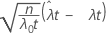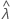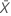# Methods and formulas for 1-Sample Poisson Rate

Select the method or formula of your choice.

## Statistics

Minitab generates the following descriptive statistics from your sample. Minitab displays a mean only if you change "length" from its default value of 1.
TermDescription
rate of occurrence
mean occurrence

The rate equals the average number of occurrences per unit length of observation. The mean is the average number of occurrences in the entire sample. If length equals 1, then rate and mean are equal.

## P-value for exact test

### Formula

The exact test for 1-Sample Poisson Rate uses the following equations to calculate p-values for the respective alternative hypotheses:
• H1: λ > λ0: p-value = P(Ss | λ = λ0) where S has a Poisson distribution with mean 0t.
• H1: λ < λ0: p-value = P(Ss | λ = λ0) where S has a Poisson distribution with mean 0t.
• H1: λλ0: Minitab uses a likelihood ratio test as follows:
Define the function G(s), which expresses the likelihood ratio test in terms of s, the total number of occurrences in the Poisson process:
• if 0 ≤ s < 0t, solve the equation G(y) = G(s) for y in the interval (0t, enλ0t];

p-value = P(Ss | λ = λ0) + P(Sy | λ = λ0)

• if s = 0t, then

p-value = 1.00

• if 0t < senλ0t, solve the equation G(y) = G(s) for y in the interval [0, 0t);

p-value = P(Sy | λ = λ0) + P(Ss | λ = λ0)

• if s > enλ0t, the test is one-sided, and

p-value = P(Ss | λ = λ0)

where S has a Poisson distribution with mean 0t.

### Notation

TermDescription
s total number of occurrences in the Poisson process
t"length" of observation
λ0hypothesized value of the population rate paramter
λtrue value of the population rate parameter
nsample size
e 2.71828, approximately

## Confidence intervals and confidence bounds for exact test

### Confidence intervals

An exact 100(1 – α)% confidence interval for the occurrence rate of a Poisson process is given by:

When you specify a value of "length", Minitab also displays a confidence interval for the mean number of occurrences. That confidence interval given by:

### Confidence bounds

When you specify a one-sided test, Minitab calculates a one-sided 100(1 – α)% confidence bound, according to the direction of the alternative hypothesis.

• If you specify a "greater than" alternative hypothesis, the exact 100(1 – α)% lower bound of the rate is given by:

The exact 100(1 – α)% lower bound of the mean is given by:

• If you specify a "less than" alternative hypothesis, the exact 100(1 – α)% upper bound of the rate is given by:

The exact 100(1 – α)% upper bound of the mean is given by:

### Notation

TermDescription
stotal number of occurrences in the Poisson process
t"length" of observation
λtrue value of the population rate
μtrue value of the population mean
Χ2(p, x)upper x percentile point of the Χ2 distribution with p degrees of freedom, where 0 < x < 1.
αalpha-level for the 100(1–α)% confidence interval
nsample size

## P-value for normal approximation

Normal approximations are valid if the total number of occurrences is greater than 10.

### Formula

The hypothesis test based on a normal approximation for 1-Sample Poisson Rate uses the following p-value equations for the respective alternative hypotheses:

### Notation

TermDescription
Zt "length" of observation
λ 0 hypothesized value of the population rate parameter
λ true value of the population rate parameterobserved value of the sample rate statistic
n sample size

## Confidence intervals and confidence bounds for normal approximation

### Confidence intervals

An 100(1 – α)% confidence interval, based on a normal approximation, for the occurrence rate of a Poisson process is given by:

When you specify a value of "length", Minitab also displays a confidence interval for the mean number of occurrences. That confidence interval is given by:

### Confidence bounds

When you specify a one-sided test, Minitab calculates a one-sided 100(1 – α)% confidence bound, according to the direction of the alternative hypothesis.
• If you specify a "greater than" alternative hypothesis, the exact 100(1 – α)% lower bound of the rate is given by:

If you specify a value of "length", the exact 100(1-α)% lower bound of the mean is given by:

• If you specify a "less than" alternative hypothesis, the exact 100(1 – α)% upper bound of the rate is given by:

If you specify a value of "length", the exact 100(1 – α)% upper bound of the mean is given by:

### Notation

TermDescription
s total number of occurrences in the Poisson process
t"length" of observation
λtrue value of the population rate
μtrue value of the population mean
Zxupper x percentile point of the standard normal distribution, where 0 < x < 1.
αalpha-level for the 100(1–α)% confidence intervalmean number of occurrences in the sample
nsample size
By using this site you agree to the use of cookies for analytics and personalized content.  Read our policy